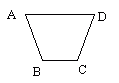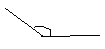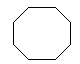Email us to get an instant 20% discount on highly effective K-12 Math & English kwizNET Programs!

#### Online Quiz (WorksheetABCD)

Questions Per Quiz = 2 4 6 8 10

### Grade 3 - Mathematics8.19 Geometry Review Test

 Q 1: This polygon has _____angles423 Q 2: Area of a square =2 x sideside x sideside + side Q 3: What shape has two circle faces?Answer: Q 4: The figure shows regular triangle which is also called __________ triangle.Answer: Q 5: The figure shown is a regularpentagonoctagonhexagon Q 6: What is the shape with 12 faces, each formed by an regular pentagon?dodecahedrondocahedron12hedrons Q 7: An angle that is greater than a right angle is calleda great angleobtuse angleacute angle Q 8: The figure shown is a regularoctagonpentagonhexagon Question 9: This question is available to subscribers only! Question 10: This question is available to subscribers only!